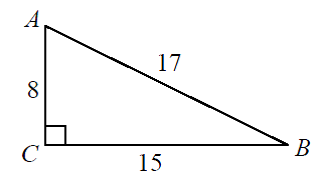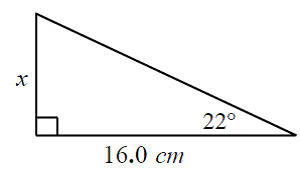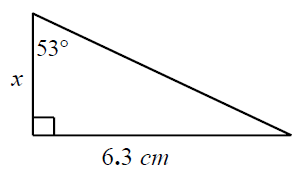# Use tangent ratio to calculate angles and sides (Tan = o / a)#### Everything You Need in One Place

Homework problems? Exam preparation? Trying to grasp a concept or just brushing up the basics? Our extensive help & practice library have got you covered.#### Learn and Practice With Ease

Our proven video lessons ease you through problems quickly, and you get tonnes of friendly practice on questions that trip students up on tests and finals.#### Instant and Unlimited Help

Our personalized learning platform enables you to instantly find the exact walkthrough to your specific type of question. Activate unlimited help now!

0/1
##### Intros
###### Lessons
1. What are sine, cosine and tangent?
0/7
##### Examples
###### Lessons
1. Determine each tangent ratio using a calculator
i) $\tan 70^\circ$
ii) $\tan-134^\circ$
1. Determine the angle to the nearest degree using a a calculator
i) $\tan \theta = 0.64$
ii) $\tan \theta = -1.45$
1. Determine the angles and sides using Tangent
1. Find angle A and B:2. Find the value of "x" using tangent3. Find the value of "x" using tangent2. Draw and label a right triangle to illustrate tangent ratio, then calculate the angle.
1. $\tan \theta = {5 \over 9}$
###### Topic Notes
Tangent ratio is yet another trigonometric ratio for right-angled triangles. Tangent ratio is the ratio of opposite side to adjacent side of a right triangle. Same as the sine and cosine ratios, tangent ratios can be used to calculate the angles and sides of right angle triangles.